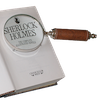#### You may also like### Number Round Up

Arrange the numbers 1 to 6 in each set of circles below. The sum of each side of the triangle should equal the number in its centre.### Number Detective

Follow the clues to find the mystery number.### Grouping Goodies

Pat counts her sweets in different groups and both times she has some left over. How many sweets could she have had?

# Venn Diagrams

##### Age 5 to 11Challenge Level

Thank you to everybody who sent in their answers to these questions.

Tetsu from St Michael's International School in Japan sent us some ideas about how a Venn diagram might work. Tetsu noticed that there are two circles that overlap, and the middle part where they overlap is where we write numbers that are both even and are multiples of 5. Well done for writing down what you could see and what you wondered when you looked at the diagram!

Harry from Copthorne Prep School in the UK noticed that only the numbers that met the description of the circle were allowed in. He worked out that if they met the descriptions of both circles then they went into the crossover part, and if they didn't meet the description of either circle then they had to stay outside. He made this video explaining how to put the numbers into the Venn diagrams:

Well done, Harry! We've also uploaded an image of Harry's solutions.

Lyra, Giselle and Taylor from Westridge in the USA explained their ideas for the first three Venn diagrams:

You would put the not even numbers and multiples of 5 in the right circle (multiples of 5), put the both even and multiples of 5 numbers in the middle (where the circles combine), put the even numbers that are not multiples of 5 in the left circle, and you would put the numbers that are neither even or multiples of 5 outside of the circle.

In the second diagram, put the numbers that are less than 20 that are not odd in the right circle, the numbers that are odd and not less than 20 you put in the left circle, put the numbers that are odd and less than 20 in the middle (where the circles combine), and put the numbers that are neither odd or less than 20 outside of the circles.

In the third diagram, the labels should be: the left one should be labeled "odd", and the right one should be labeled "higher than 20".

Thank you all for explaining this so clearly.

Abbie, also from Westridge, noticed something interesting about the last diagram:

Also, for the diagram that says "even numbers" and "odd numbers", there can't be an even AND odd number unless you are going to count zero. That would be a trick question to those who don't know their numbers well enough yet.

That's a really good idea, Abbie - I wonder if we would count zero as even number, an odd number, both or neither!

Haleema from Pierrepont Gamston Primary School also had an idea about the last diagram:

The thing I noticed on the last one was that if the labels are even and odd numbers there can't be anything in the middle. I don't think a Venn diagram should have been used for that. Maybe you could draw circles and label them but there just shouldn't be a middle section.

This is a good idea, Haleema - if we drew a diagram with two circles that didn't overlap, would that still be a Venn diagram or would it be a different type of diagram? I wonder if there are any other types of diagram we could use for two types of numbers where there isn't any overlap.

Finally, Sarah from the ABQ Seeb International School in Oman sent us this video explaining how to put the numbers into the first Venn diagram and into her own Venn diagram:

Thank you for that very clear explanation, Sarah.